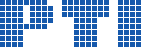Polish Information Processing Society# Computing the minimal solutions of finite fuzzy relation equations on lineal carriers

## Juan Carlos Díaz-Moreno, Jesús Medina, Esko Turunen

Citation: Position Papers of the 2016 Federated Conference on Computer Science and Information Systems, M. Ganzha, L. Maciaszek, M. Paprzycki (eds). ACSIS, Vol. 9, pages 1923 ()

Full text

Abstract. Fuzzy relation equation is a important tool for managing and modeling uncertain or imprecise datasets, which has useful applied to, e.g. approximate reasoning, time series forecast, decision making, fuzzy control, etc. This paper introduces a mechanism in order to compute the minimal solutions of a considered general fuzzy relation equation. The corresponding algorithms and different illustrative examples have also presented.

### References

1. W. Bandler and L. Kohout. Semantics of implication operators and fuzzy relational products. Int. J. Man-Machine Studies, 12:89–116, 1980.
2. E. Bartl. Minimal solutions of generalized fuzzy relational equations: Probabilistic algorithm based on greedy approach. Fuzzy Sets and Systems, 260(0):25 – 42, 2015.
3. R. Bělohlávek. Fuzzy Relational Systems: Foundations and Principles. Kluwer Academic Publishers, 2002.
4. L. Chen and P. Wang. Fuzzy relation equations (ii): The branch-point-solutions and the categorized minimal solutions. Soft Computing–A Fusion of Foundations, Methodologies and Applications, 11:33–40, 2007.
5. M. E. Cornejo, J. Medina, and E. Ramírez-Poussa. Multi-adjoint algebras versus non-commutative residuated structures. International Journal of Approximate Reasoning, 66:119–138, 2015.
6. B. De Baets. Analytical solution methods for fuzzy relation equations. In D. Dubois and H. Prade, editors, The Handbooks of Fuzzy Sets Series, volume 1, pages 291–340. Kluwer, Dordrecht, 1999.
7. J. C. Díaz-Moreno, J. Medina, and M. Ojeda-Aciego. On basic conditions to generate multi-adjoint concept lattices via galois connections. International Journal of General Systems, 43(2):149–161, 2014.
8. J. C. Díaz-Moreno, J. Medina, and E. Turunen. Minimal solutions of general fuzzy relation equations on linear carriers. an algebraic characterization. Fuzzy Sets and Systems, 2016.
9. P. Hájek. Metamathematics of Fuzzy Logic. Trends in Logic. Kluwer Academic, 1998.
10. J.-L. Lin, Y.-K. Wu, and S.-M. Guu. On fuzzy relational equations and the covering problem. Information Sciences, 181(14):2951–2963, 2011.
11. E. Sanchez. Resolution of composite fuzzy relation equations. Informa- tion and Control, 30(1):38–48, 1976.
12. B.-S. Shieh. Solution to the covering problem. Information Sciences, 222(0):626–633, 2013.
13. E. Shivanian. An algorithm for finding solutions of fuzzy relation equations with max-lukasiewicz composition. Mathware and Soft Computing, 17:15–26, 2010.
14. E. Turunen. On generalized fuzzy relation equations: necessary and sufficient conditions for the existence of solutions. Acta Universitatis Carolinae. Mathematica et Physica, 028(1):33–37, 1987.
15. Q.-Q. Xiong and X.-P. Wang. Solution sets of fuzzy relational equations on complete Brouwerian lattices. Information Sciences, 177(21):4757– 4767, 2007.
16. Q.-Q. Xiong and X.-P. Wang. Fuzzy relational equations on complete Brouwerian lattices. Information Sciences, 193(0):141–152, 2012.
17. C.-T. Yeh. On the minimal solutions of max-min fuzzy relational equations. Fuzzy Sets and Systems, 159(1):23–39, 2008.
18. Z. Zahariev. http://www.mathworks.com/matlabcentral,fuzzy-calculus-core-fc2ore, 2010.